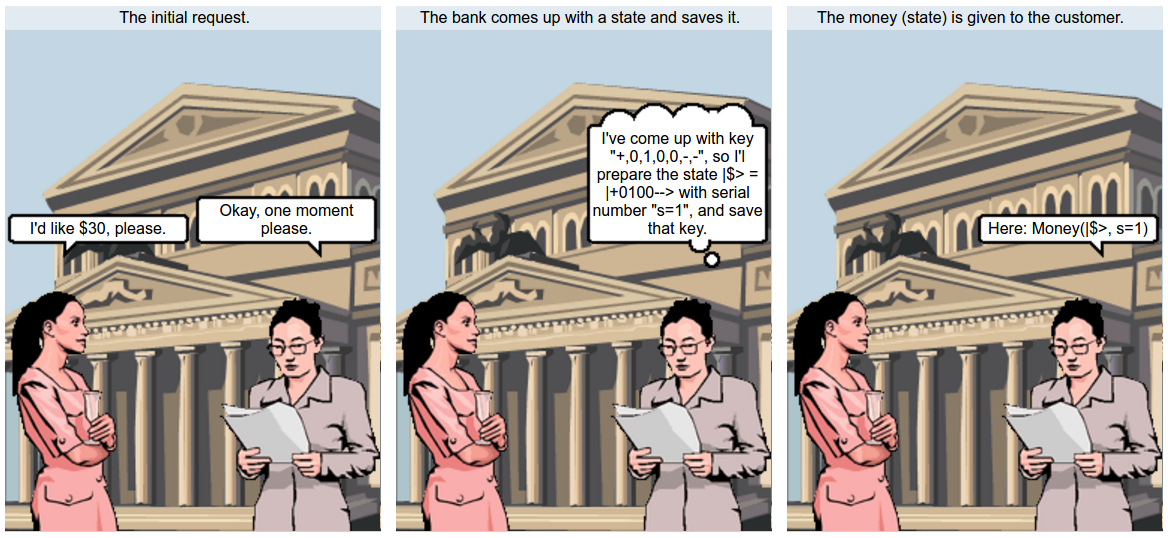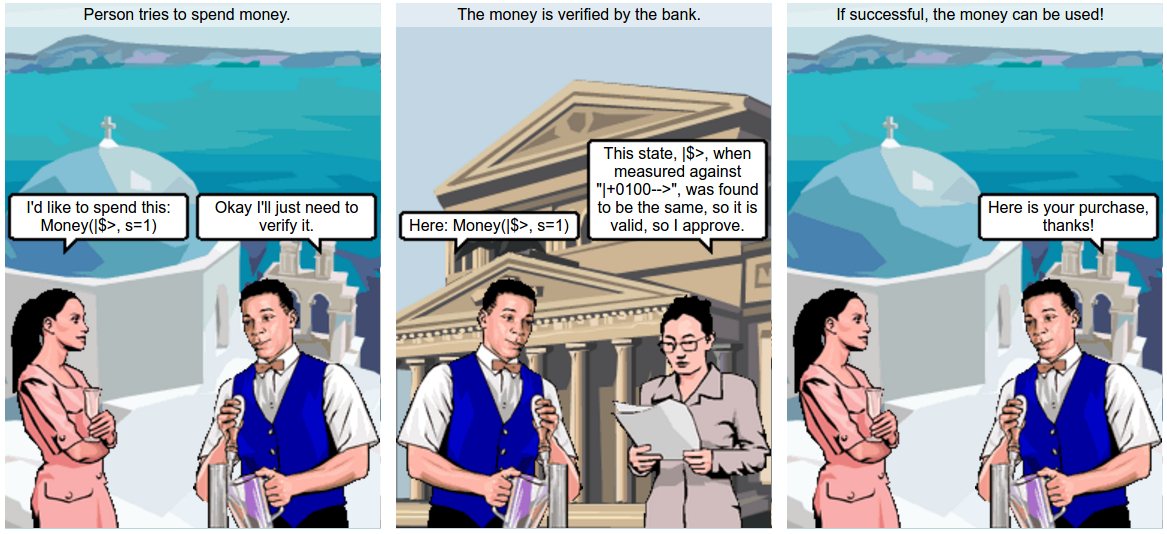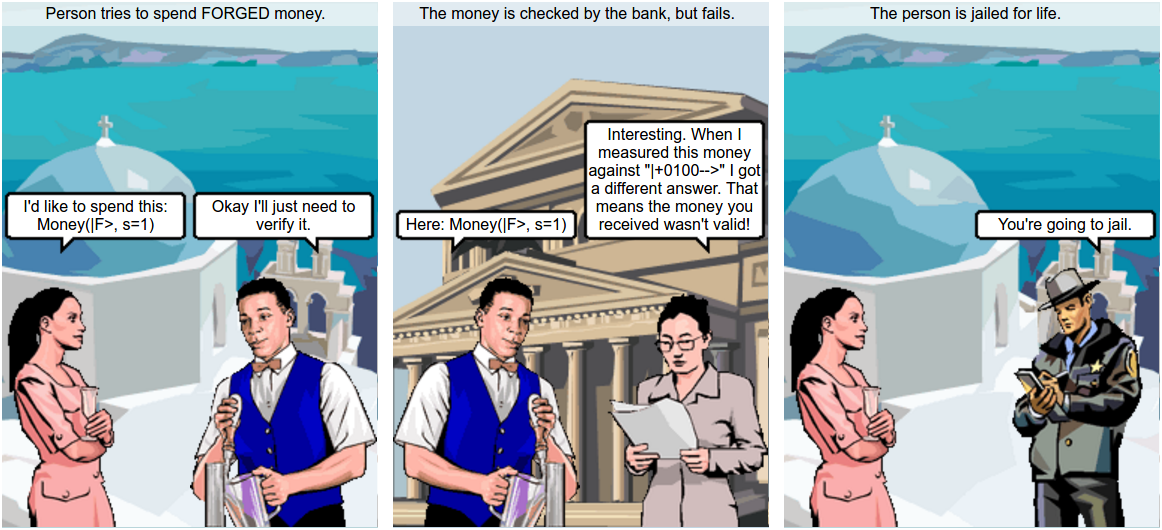## Forging quantum money (part 1 of 3)

Posted on January 6, 2017 by Noon van der Silk

Due to the release of (yet another) Python framework for quantum simulation ProjectQ, I was inspired to revisit the paper An adaptive attack on Wiesner’s quantum money from a few years back.

This post will form the first post of a three-part series on the paper, and the background necessary to understand the part of it I’ll cover. We’ll learn about:

1. Wiesner’s scheme for quantum money, and why it was considered secure (this post),
2. An attack on Wiesner’s money scheme that shows it is not secure (with an aside into quantum bomb testing!),
3. and, A modification of Wiesner’s scheme that resists this attack.

# Wiesner’s scheme

Wiesner’s Quantum Money scheme is one of the earliest ideas in quantum computing. The fundamental idea is that (unknown) quantum states cannot be copied arbitrarily and hence make an “unforgable” form of money: it shouldn’t be possible to duplicate a “quantum” bill.

Money, in Wiesner’s scheme, is created by the bank. The bank holds a serial number and a secret “key” that the bank uses to verify each note that it hands out. When given a note, the bank can determine if it is valid by referring back to this secret key.

The important steps are:

4. Yield a bank note to the customer with the given serial number s and the embedded quantum state |⟩.Money verification: To verify a given piece of money, the bank proceeds as follows: 1. Look up the key for the provided serial number s, 2. Measure each qubit in the basis that the we know it was created from. 3. If the money state hasn’t changed, then we will measure it to be in the same state that we created it, and we can say that it is valid.4. If the money state has changed, then there is a chance that the measurement results will disagree, and we can be sure that it has been tampered with.5. In the case that validation succeeds, the money state is returned to the person requesting validation, and in the case that the validation fails, the money is destroyed. Having the same money state returned, instead of a new one each time validation succeeds, is critical to the success of the forging approach of arXiv:1404.1507. ## Standard analysis of Wiesner’s scheme Let’s look at an example. Suppose we have withdrawn some money from the bank, and the state we’ve been given (but can’t see) is \begin{aligned} |\\rangle &= |+100--\rangle. \end{aligned} There are six qubits, and we can see the bases that each has been prepared in, but if we’re simply the customer we don’t know this information. Our goal is to create a state |F that the bank will also verify as valid. Noting that if we measure either | + ⟩ or | − ⟩ in the computational basis, we’ll get |0⟩ with 50% probability or |1⟩ with 50% probability, one approach is simply to build |F by the following technique: 1. Measure each qubit indepnedently in the computational basis, and obtain some measurement outcome x ∈ {0, 1}. 2. In this way, build up |F from states |x. In our example, we can see that this will work 50% of the time for the first qubit of |⟩, 100% of the time for the 2nd, 3rd and 4th qubits, and again 50% of the time for the last two qubits. So for this state, this approach will succeed with probability $\left( \frac{1}{2} \right)^3 = \frac{1}{8}$. Pretty bad odds.

In an earlier paper, Molina Vidick and Watrous show that in a model where the attacker doesn’t interact with the bank after receiving the note, the best attack that one can mount results in a success probability of $\left(\frac{3}{4}\right)^n$, where n is the number of qubits in the money state. This is better than my approach here, but if we set n to be of modest size, say n = 10, then this approach will succeed at most 5 times out of 100; still not particularly good. If we had 100 twenty dollar notes, we could attempt to forge them, and we’d end up with a total of 2 × 5 × 20 = $200 instead of the original$2, 000 we started with.

# Summary

We’ve seen that Wiesner’s original scheme for quantum money doesn’t appear to be forgable with our first ideas. In the next post we’ll learn about a very cool technique in quantum mechanics, the Elitzur-Vaidman bomb tester, and then we’ll see how it can be used to beat Wiesner’s scheme!

1. A one-qubit basis is a set of states such that any state involving one qubit can be written as a linear combination of either of the elements in the basis.